# CD Set Data to Model VI

LabVIEW 2018 Control Design and Simulation Module Help

Edition Date: March 2018

Part Number: 371894J-01

»View Product InfoDownload Help (Windows Only)

Owning Palette: Model Information VIs

Requires: Control Design and Simulation Module

Sets the given values to the system model. Wire data to the State-Space Model In input to determine the polymorphic instance to use or manually select the instance.

Use the pull-down menu to select an instance of this VI.

 Select an instance CD Set Data to Model (State-Space)CD Set Data to Model (Transfer Function)CD Set Data to Model (Zero-Pole-Gain)

## CD Set Data to Model (State-Space)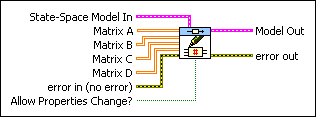State-Space Model In contains a mathematical representation of and information about the system for which this VI modifies data.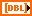Matrix A is the system matrix that describes the dynamics of the states of the system. If you do not wire an input to Matrix A, this VI uses the matrix A from the State-Space Model In input.Matrix B is the input matrix of the system that relates the inputs to the states. If you do not wire an input to Matrix B, this VI uses the matrix B from the State-Space Model In input.Matrix C is the output matrix that relates the outputs to the states. If you do not wire an input to Matrix C, this VI uses the matrix C from the State-Space Model In input.Matrix D is the transmission matrix that relates the inputs of the systems to its outputs. If you do not wire an input to Matrix D, this VI uses the matrix D from the State-Space Model In input.error in describes error conditions that occur before this node runs. This input provides standard error in functionality.Allow Properties Change? specifies if the new data values in Matrix A, Matrix B, Matrix C, or Matrix D change the number of inputs, outputs, or states in the system model. The default value is FALSE.Model Out is the modified form of State-Space Model In with the values that the Matrix A, Matrix B, Matrix C, and Matrix D inputs specify. The properties are the same as that of State-Space Model In. To access and modify the data in the model, use the Model Information VIs.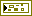error out contains error information. This output provides standard error out functionality.

## CD Set Data to Model (Transfer Function)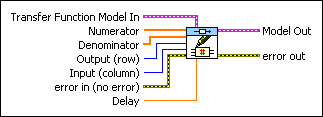Transfer Function Model In contains a mathematical representation of and information about the system for which this VI modifies data.Numerator represents the numerator, in ascending order, of the transfer function as Output (row) and Input (column) specify.Denominator represents the denominator, in ascending order, of the transfer function as Output (row) and Input (column) specify.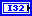Output (row) specifies the output, or row, number of the transfer function in which this VI sets data. This number is necessary when the model represents a MIMO system. The index is zero-based. The default is zero.Input (column) specifies the index number of the input column of the transfer function in which this VI sets data. This number is necessary when the model represents a MIMO system. The index is zero-based. The default is zero.error in describes error conditions that occur before this node runs. This input provides standard error in functionality.Delay is the transport time delay between the Output (row) and Input (column) pair. The default is Nan. Refer to the LabVIEW Control Design User Manual for more information about delays.Model Out is the modified form of Transfer Function In with the values as the Numerator, Denominator, and Delay inputs specify. The properties are the same as that of Transfer Function Model In. To access and modify the data in the model, use the Model Information VIs.error out contains error information. This output provides standard error out functionality.

## CD Set Data to Model (Zero-Pole-Gain)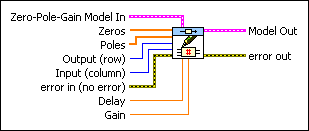Zero-Pole-Gain Model In contains a mathematical representation of and information about the system for which this VI modifies data.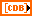Zeros represents the array of zeros, in ascending order, of the system that Output (row) and Input (column) specify.Poles represents the array of poles, in ascending order, of the system that Output (row) and Input (column) specify.Output (row) specifies the output, or row, number of the transfer function (in zero-pole-gain form) in which this VI returns data. This number is necessary when the model represents a MIMO system. The index is zero-based. The default value is zero.Input (column) specifies the index number of the input column of the transfer function (in zero-pole-gain form) in which this VI returns data. This number is necessary when the model represents a MIMO system. The index is zero-based. The default is zero.error in describes error conditions that occur before this node runs. This input provides standard error in functionality.Delay is the transport time delay between the Output (row) and Input (column) pair. The default is Nan. Refer to the LabVIEW Control Design User Manual for more information about delays.Gain is the scalar gain of the system that Output (row) and Input (column) specify.Model Out is the modified form of Zero-Pole-Gain Model In with the values that the Zeros, Poles, Delay, and Gain inputs specify. The properties are the same as that of Zero-Pole-Gain Model In. To access and modify the data in the model, use the Model Information VIs.error out contains error information. This output provides standard error out functionality.